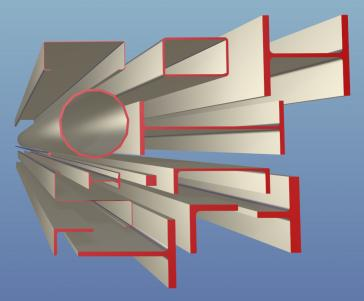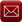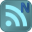You have downloaded this file 0 times in the last 24 hours, limit is 100.

## AISC 14.1 Properties Viewer.xls This location is for Registered Users Only.Perhaps you need to login or register.

Description:This workbook is based on the "AISC Shapes Database" Version 14.1 (October 2013), and has the same look as the ones that I did based on earlier AISC shapes database/manual versions.

Here is a link to the AISC.org website where the source database file can be found:
http://www.aisc.org/content.aspx?id=2868

NOMENCLATURE FOR AISC VERSION 14.1 MEMBER PROPERTIES AND DIMENSIONS:

A = Cross-sectional area of member (in.^2)
d = Depth of member, parallel to Y-axis (in.)
h = Depth of member, parallel to Y-axis (in.)
tw = Thickness of web of member (in.)
bf = Width of flange of member, parallel to X-axis (in.)
b = Width of member, parallel to X-axis (in.)
tf = Thickness of flange of member (in.)
k = Distance from outer face of flange to web toe of fillet (in.)
k1 = Distance from web centerline to flange toe of fillet (in.)
T = Distance between fillets for wide-flange or channel shape = d(nom)-2*k(det) (in.)
gage = Standard gage (bolt spacing) for member (in.)  (Note: gages for angles are available by viewing comment box at cell K18.)
Ix = Moment of inertia of member taken about X-axis (in.^4)
Sx = Elastic section modulus of member taken about X-axis (in.^3)
rx = Radius of gyration of member taken about X-axis (in.) = SQRT(Ix/A)
Iy = Moment of inertia of member taken about Y-axis (in.^4)
Sy = Elastic section modulus of member taken about Y-axis (in.^3)
ry = Radius of gyration of member taken about Y-axis (in.) = SQRT(Iy/A)
Zx = Plastic section modulus of member taken about X-axis (in.^3)
Zy = Plastic section modulus of member taken about Y-axis (in.^3)
rts = SQRT(SQRT(Iy*Cw)/Sx) (in.)
xp = horizontal distance from designated member edge to plastic neutral axis (in.)
yp = vertical distance from designated member edge to plastic neutral axis (in.)
ho = Distance between centroid of flanges, d-tf (in.)
J = Torsional moment of inertia of member (in.^4)
Cw = Warping constant (in.^6)
C = Torsional constant for HSS shapes (in.^3)
a = Torsional property, a = SQRT(E*Cw/G*J) (in.)
E = Modulus of elasticity of steel = 29,000 ksi
G = Shear modulus of elasticity of steel = 11,200 ksi
Wno = Normalized warping function at a point at the flange edge (in.^2)
Sw = Warping statical moment at a point on the cross section (in.^4)
Qf = Statical moment for a point in the flange directly above the vertical edge of the web (in.^3)
Qw = Statical moment at the mid-depth of the section (in.^3)
x(bar) = Distance from outside face of web of channel shape or outside face of angle leg to Y-axis (in.)
y(bar) = Distance from outside face of outside face of flange of WT or angle leg to Y-axis (in.)
eo = Horizontal distance from the outer edge of a channel web to its shear center (in.) = (approx.) tf*(d-tf)^2*(bf-tw/2)^2/(4*Ix)-tw/2
xo = x-coordinate of shear center with respect to the centroid of the section (in.)
yo = y-coordinate of shear center with respect to the centroid of the section (in.)
ro(bar) = Polar radius of gyration about the shear center = SQRT(xo^2+yo^2+(Ix+Iy)/A) (in.)
H = Flexural constant, H = 1-(xo^2+yo^2)/ro(bar)^2)
LLBB = Long legs back-to-back for double angles
SLBB = Short legs back-to-back for double angles
h(flat) = The workable flat (straight) dimension along the height, h (in.)
b(flat) = The workable flat (straight) dimension along the width, b (in.)
A(surf) = The total surface area of a rectangular or square HSS section (ft.^2/ft.)
STD = Standard weight (Schedule 40) pipe section
XS = Extra strong (Schedule 80) pipe section
XXS = Double-extra strong pipe section

Calculation Reference
AISC MEMBER PROPERTIES AND DIMENSIONS
Steel Design
Structural Steel

Submitted By:
Alex Tomanovich (ATomanovich)
Submitted On:
26 Nov 2019
File Size:
643.50 Kb
239
File Version:
1.1
File Author:
Alex Tomanovich
Rating:Total Votes:5
ATomanovich 12 days ago
In my AISC 14.1 Properties Viewer.xls spreadsheet, it was brought to my attention that the drop down pick box in Cell N18 for double angles was not formatted correctly to include the entire list of shapes that was included off to the right of the main page. This has now been corrected and the current version is 1.1.
rahulmenon 5 years ago
excellent but this one is amazing too
http://www.aisc.org/content.aspx?id=38848

We have 419 guests and 3 members onlineEmail (preferred method of contact)US +1 617 5008224EU +44 113 8152220